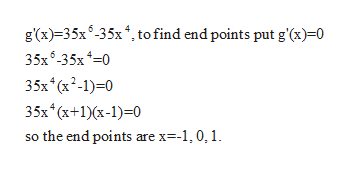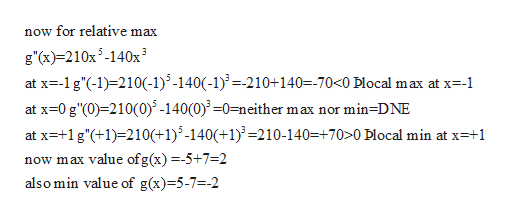# Let g(x) = 5x7 − 7x5Find the relative maximum and minimum values of g. Also find the intervals on which the graph of g is concave upward. Enter the values of the endpoints in the appropriate blanks and enter DNE in any unused answer blanks. Finally, sketch the graph of g, following the directions above, and bring it to your discussion section the day this assignment is due.Relative maximum values, with the x-values in increasing order:g( ) = g( ) = Relative minimum values, with the x-values in increasing order:g( ) = g( ) =The graph is concave upward on the following interval(s):(−∞, ∞)(−∞, a)    (−∞, a](a, ∞)[a, ∞)(−∞, a) ∪ (b, ∞)(−∞, a] ∪ [b, ∞)(−∞, a) ∪ (b, c)(a, b) ∪ (c, ∞)(a, b)[a, b]None of the above.a =  b =  c =

Question
15 views
Let g(x) = 5x7 − 7x5

Find the relative maximum and minimum values of g. Also find the intervals on which the graph of g is concave upward. Enter the values of the endpoints in the appropriate blanks and enter DNE in any unused answer blanks. Finally, sketch the graph of g, following the directions above, and bring it to your discussion section the day this assignment is due.

Relative maximum values, with the x-values in increasing order:
g( ) =
g( ) =

Relative minimum values, with the x-values in increasing order:
g( ) =
g( ) =

The graph is concave upward on the following interval(s):

(−∞, ∞)
(−∞, a)

(−∞, a]
(a, ∞)
[a, ∞)
(−∞, a) ∪ (b, ∞)
(−∞, a] ∪ [b, ∞)
(−∞, a) ∪ (b, c)
(a, b) ∪ (c, ∞)
(a, b)
[a, b]
None of the above.

a =

b =

c =

check_circle

Step 1

first derivative.

Step 2

calculation for end points.help_outlineImage Transcriptioncloseg'(x)-35x6-35x,to find end points put g'(x)0 35x-35x-0 35x (x2-1)0 35x (x+1)(x-1)-0 the end points are x-1, 0,1 fullscreen
Step 3

the values of x for relative max , relative min a...help_outlineImage Transcriptionclosenow for relative max g'(x)-210x5-140x at x-1g'(-1)-210(-1)5-140(-1)?=-210+140=70<0 Dlocal max at x-1 at x0 g'(0)-210(0)'-140(0)=0=neither max nor min=DNE at x+1g"(+1)-210(+1)-140(+1)=210-140-+70>0 Dlocal min at x-+1 now max value ofg(x) =-5+7=2 also min value of g(x)=5-7=-2 fullscreen

### Want to see the full answer?

See Solution

#### Want to see this answer and more?

Solutions are written by subject experts who are available 24/7. Questions are typically answered within 1 hour.*

See Solution
*Response times may vary by subject and question.
Tagged in
MathCalculus

### Derivative